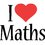# MatriX

Find two non zero matrix such that :

# AB = BA = 0Note by Syed Baqir
5 years, 2 months ago

This discussion board is a place to discuss our Daily Challenges and the math and science related to those challenges. Explanations are more than just a solution — they should explain the steps and thinking strategies that you used to obtain the solution. Comments should further the discussion of math and science.

When posting on Brilliant:

• Use the emojis to react to an explanation, whether you're congratulating a job well done , or just really confused .
• Ask specific questions about the challenge or the steps in somebody's explanation. Well-posed questions can add a lot to the discussion, but posting "I don't understand!" doesn't help anyone.
• Try to contribute something new to the discussion, whether it is an extension, generalization or other idea related to the challenge.

MarkdownAppears as
*italics* or _italics_ italics
**bold** or __bold__ bold
- bulleted- list
• bulleted
• list
1. numbered2. list
1. numbered
2. list
Note: you must add a full line of space before and after lists for them to show up correctly
paragraph 1paragraph 2

paragraph 1

paragraph 2

[example link](https://brilliant.org)example link
> This is a quote
This is a quote
    # I indented these lines
# 4 spaces, and now they show
# up as a code block.

print "hello world"
# I indented these lines
# 4 spaces, and now they show
# up as a code block.

print "hello world"
MathAppears as
Remember to wrap math in $$ ... $$ or $ ... $ to ensure proper formatting.
2 \times 3 $2 \times 3$
2^{34} $2^{34}$
a_{i-1} $a_{i-1}$
\frac{2}{3} $\frac{2}{3}$
\sqrt{2} $\sqrt{2}$
\sum_{i=1}^3 $\sum_{i=1}^3$
\sin \theta $\sin \theta$
\boxed{123} $\boxed{123}$

Sort by:

Well, this one works

$A=B=\begin{pmatrix} 0 & 1 \\ 0 & 0 \end{pmatrix}$

and this one too

$A=\begin{pmatrix} 0 & 1 \\ 0 & -1 \end{pmatrix}\quad and\quad B=\begin{pmatrix} 1 & 1 \\ 0 & 0 \end{pmatrix}$

there's lots of such matrices that have this property.

- 5 years, 2 months ago

But our matrix must be non- zero

- 5 years, 2 months ago

You mean non-zero determinant? That will take me a little longer.

- 5 years, 2 months ago

I mean matrix in which none of the elements are 0

- 5 years, 2 months ago

Here you go

$A=\begin{pmatrix} 1 & 1 \\ 1 & 1 \end{pmatrix}\quad and\quad B=\begin{pmatrix} -1 & 1 \\ 1 & -1 \end{pmatrix}$

As I said, finding two matrices with non-zero determinants will take a bit longer. Normally it shouldn't be possible, but I've heard of algebras where this is possible, i.e., the elements aren't restricted to reals. You didn't specify if the elements had to be reals or even complex.

- 5 years, 2 months ago

You are correct but I think B must be like this:

B = $\begin{pmatrix} 1 & -1 \\ -1 & 1 \end{pmatrix}$

- 5 years, 2 months ago

Well obviously it can work both ways.

- 5 years, 2 months ago

But sir can you tell us how you got the answer I mean the method ?

- 5 years, 2 months ago

Trial and error. But it's a LOT harder problem if the matrices have non-zero determinants! Don't ask me tonight, I'm going to sleep pretty soon!

- 5 years, 2 months ago

hehe, But I will ask you tomorrow :P,

Any way nice solution I was using brute force method .

If you have time, dont forget to enlighten this note with brilliant solution !!

- 5 years, 2 months ago

I will sleep on a brilliant solution tonight! Good night!

- 5 years, 2 months ago

Good Night !!

- 5 years, 2 months ago

The product of the determinants of the 2 matrix has the same value as the determinant of their product, so either must have 0 determinant.

- 5 years, 2 months ago

But the elements must not contain any 0

- 5 years, 2 months ago

Yeah, I just said about if the determinant is 0. In fact, if exactly one 0 exist, a 2x2 matrix wouldn't have zero determinant.

- 5 years, 2 months ago

Can you show us the method ?

- 5 years, 2 months ago

I guess you need to consider 2 matrices. And then, you multiply them (I told that for 2x2 matrices!!!), next you calculate the determinant of the result. I hope that you can do it yourself using this.

- 5 years, 2 months ago

So it's actually simple problem if we are asked to find one each with non-zero determinants: say that it's impossible.

- 5 years, 2 months ago

lol, then you will get impossible marks in the result !!!

- 5 years, 2 months ago